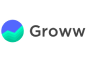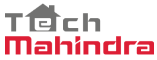New update is available. Click here to update.
Topics

# Delete middle element from stack

Easy0/40
Average time to solve is 15m+3 more companies

## Problem statement

You are having a stack "ARR" of size 'N+1', your task is to delete the middlemost element so that the size of resulting stack is 'N'.

A stack is a linear data structure where both insertion and deletion of elements take place at the top. It follows FILO (First In Last Out) or LIFO (Last In First Out) approaches. Books piled on top of each other is an example of a stack, where you can only remove a single book at a time, which is at the top of the stack. Likewise, you can only add a single book at a time, on the top of the stack only.

Example :-
``````INPUT : ARR [ ] = [ 1 , 2 , 3 , 4 , 5 ] , N = 4
OUTPUT: ARR [ ] = [ 1 , 2 , 4,  5 ]

The above example contains an odd number of elements, hence the middle element is clearly the (N+1) / 2th element, which is removed from the stack in the output.

INPUT : ARR [ ] = [ 5, 6, 7, 8 ] , N = 3
OUTPUT: ARR [ ] = [ 5, 7, 8 ]

The above example contains an even number of elements, so out of the two middle elements, we consider the one which occurs first. Hence, the middle element would be ((N+1) / 2 - 1) element, which is 6 and is removed from the stack in the output.
``````
Detailed explanation ( Input/output format, Notes, Images )
Constraints:
``````1 <= T <= 100
1 <= N+1 <= 3000
0 <= data <= 10^9

Where ‘T’ is the number of test cases, ‘N+1’ is the number of elements in the input Stack. ‘data’ is the value of each element in the stack.

Time limit: 1 second
``````
Sample Input 1:
``````2
4
1 2 3 4 5
7
83 74 67 49 94 8 11 1
``````
Sample Output 1:
``````1 2 4 5
83 74 67 94 8 11 1
``````

#### Explanation for Sample 1:

``````In the 1st testcase, there are an odd number of elements, hence the middle element is clearly the (N+1) / 2th element which is 3, and is removed from the stack in the output.

In the 2nd testcase, there are an odd number of elements, hence the middle element is clearly the (N+1) / 2th element which is 49, and is removed from the stack in the output.
``````
Sample Input 2:
``````3
1
5 10
4
1 3 4 2 7
5
9 5 2 7 8 6
``````
Sample Output 2:
``````10
1 3 2 7
9 5 7 8 6
``````Console